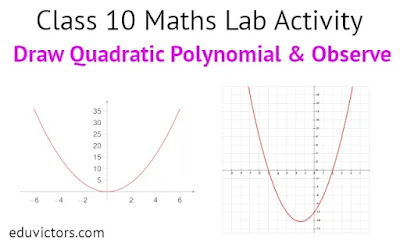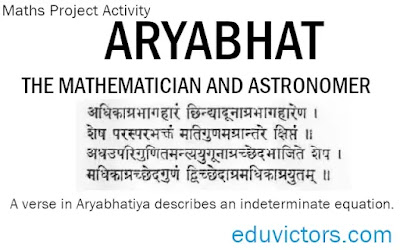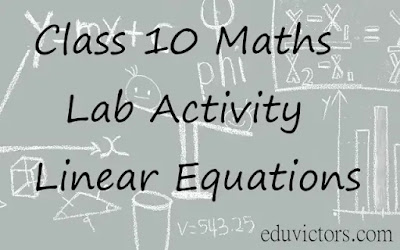## Pages

Showing posts with label class10-maths-labactivity. Show all posts
Showing posts with label class10-maths-labactivity. Show all posts

# CBSE Class 10 Maths Lab Activity - Draw Quadratic Polynomial and Observe

OBJECTIVE: To draw the graph of a quadratic polynomial and observe.

(i) The shape of the curve when the coefficient of x² is positive.

(ii) The shape of the curve when the coefficient of x² is negative.

(iii) Its number of zeroes.

MATERIAL REQUIRED

Cardboard, graph paper, ruler, pencil, eraser, pen, adhesive.# Class 10 Maths Lab Activity: Verify Pythagoras Theorem

OBJECTIVE:

To verify Pythagoras Theorem

Pythagoras theorem is a fundamental relation in Euclidean geometry among the three sides of a right triangle.

It states that the area of the square whose side is the hypotenuse is equal to the sum of the areas of the squares on the other two sides.# Class 10 Maths Activity- To verify that the lengths of tangents to a circle from some external point are equal.

OBJECTIVE
To verify that the lengths of tangents to a circle from some external point are equal.

MATERIAL REQUIRED
Glazed papers of different colours, geometry box, sketch pen, scissors, cutter and glue

METHOD OF CONSTRUCTION
1. Draw a circle of any radius, say 3 cm, with centre O on a coloured glazed paper of a convenient size.# ARYABHAT – THE MATHEMATICIAN AND ASTRONOMER

A brief profile and his contributions

Class 10 Maths Project Activity

Objective: Write about the contributions made by the ancient Indian Mathematician, Aryabhat

## ARYABHAT – THE MATHEMATICIAN AND ASTRONOMER

Life Profile:

One of the Indian mathematicians of ancient times about which some definite information is available is Aryabhat (also called Aryabhata I ).  He was born in 476 AD in Kusumpura (now Patna) in Bihar. Aryabhata wrote many mathematical and astronomical treatise and one of his famous compilation on Mathematics and Astronomy is called Aryabhattiya. He died in 550AD# Class 10 Maths Lab Activity: To verify the distance formula by graphical method

OBJECTIVE:

To verify the distance formula by graphical method.

MATERIAL REQUIRED:

Cardboard, chart paper, graph paper, glue, pen/pencil and ruler.

METHOD OF CONSTRUCTION

1. Paste a chart paper on a cardboard of a convenient size.

2. Paste the graph paper on the chart paper.

3. Draw the axes X'OX and Y'OY on the graph paper.

4. Take two points A(a, b) and B(c,d) on the graph paper and join them to get a line segment AB [see Figure].# Class 10 Maths - Lab Activity - Linear Equations

Objective

To verify the conditions for consistency of a system of linear equations in two variables by graphical representation.

Prerequisite Knowledge

1. Plotting of points on a graph paper.

2. Condition of consistency of lines parallel, intersecting, coincident,

Materials Required

Graph papers, fevicol, geometry box, cardboard.